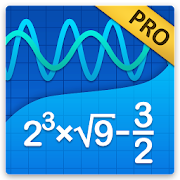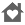# Graphing Calculator + Math PROEveryone
5,932
If you're looking for a graphing calculator app that works smoothly and seamlessly, you've found it! Graphing Calculator by Mathlab is a scientific graphing calculator integrated with algebra and is an indispensable mathematical tool for students in elementary school to those in college or graduate school, or just anyone who needs more than what a basic calculator offers. It is designed to replace bulky and costly handheld graphing calculators and works on virtually any Android phone or tablet.

Furthermore, Graphing Calculator by Mathlab displays calculations as it performs them on the high-quality display of the Android device, making it easier for the user to understand the calculations and see them clearly. This app has two great strengths. First, it acts as a fine scientific calculator, but, more than that, it displays the intermediate results of the calculations as you type. It allows the students to both watch and learn how the calculations are made and how to find the final answer. Second, the graphing ability is absolutely stunning! Not only does the calculator beautifully display the graphs, but it automatically generates the x- and y- values and displays them as well.

Video: https://youtu.be/6BR8Lv1U9kA
Help site with instructions and examples: http://help.mathlab.us
If you have a question, send email to calc@mathlab.us

PRO FEATURES
* Full screen graphs
* 9 workspaces
* Longer input and history
* Save constants, functions and expressions in the library
* Physical constants
* Internet is not required
* 30 days refund

SCIENTIFIC CALCULATOR
* Square root, cube and higher roots (hold √ key)
* Exponent or power, use x^ key, (x^2)
* Logarithms ln(), log(), log[base]()
* Trigonometric functions sin π/2, cos 30°, ...
* Hyperbolic functions sinh, cosh, tanh, ... (hold "e" key to switch)
* Inverse functions (hold direct function key)
* Complex numbers, all functions support complex arguments
* Derivatives sin x' = cos x, ... (hold x^ key)
* Scientific and engineering notation (enable in menu)
* Percent mode
* Binary, octal and hexadecimal numbers, 0b1010, 0o123, 0xABC
* Save and load history

GRAPHING CALCULATOR
* Multiple functions graphing
* Implicit functions up to 2nd degree (ellipse 2x^2+3y^2=1, etc.)
* Polar graphs (r=cos2θ)
* Parametric functions, enter each on new line (x=cos t, y=sin t)
* Function roots and critical points.
* Graph intersections
* Tracing function values and slopes
* Slide to scroll
* Pinch to zoom
* Fullscreen graphs (PRO)
* Function tables
* Save graphs as images
* Save tables as csv
* 3D graphs

FRACTION CALCULATOR
* Simple and complex fractions 1/2 + 1/3 = 5/6
* Mixed numbers, use space to enter values 3 1/2
* Use parentheses (1+2)/(3+4)=3/7

ALGEBRA CALCULATOR
* Linear equations x+1=2 -> x=1
* Quadratic equations x^2-1=0 -> x=-1,1
* Approximate roots of higher polynomials
* Systems of linear equations, write one equation per line, x1+x2=1, x1-x2=2
* Polynomial long division
* Polynomial expansion, factoring
* Linear and quadratic inequalities

PROBABILITY AND STATISTICS
* Hold n! key to enter functions, double tap dot to enter comma
* Combinations nCr(5,2)=10
* Permutations nPr(5,2)=20
* Sample A=[1 2 3 4 5]
* Sample B=[2 3 4 5 6]
* Sum sum(A) = 15
* Average avg(A) = 3
* Median median(A) = 3
* Sample variance var(A) = 2.5
* Population variance varp(A) = 2
* Standard deviation stdev(A) = 1.58..., stdevp(A) = 1.41...
* Covariance cov(A,B) = 2.5
* Correlation corr(A,B) = 1

MATRIX CALCULATOR
* Matrix and vector arithmetic operations
* Vector cross product, dot product (hold *) and norm
* Matrix determinant, inverse, transpose and trace functions

LIBRARY
* Physical constants (PRO)
* User defined constants and functions (PRO)
* Save expressions for future reference
Collapse

Review Policy
4.8
5,932 total
5
4
3
2
1

## What's New

Theme settings
Chromebooks support (new Chromebooks with Google Play)
Android 8.1 support

Exact values for trigonometric functions sin(60°) = 1/2*sqrt(3)
Polar form for complex numbers 1∠30°, hold i to enter ∠
Visual editing (beta), enable in Settings > Calculator
Collapse

Eligible for Family LibraryLearn More
Updated
March 31, 2018
Size
5.9M
Installs
100,000+
Current Version
4.14.159
Requires Android
4.0.3 and up
Content Rating
Everyone
Permissions
Offered By
Mathlab Apps, LLC
Developer
PO Box 701004 San Jose, CA 95170 United States